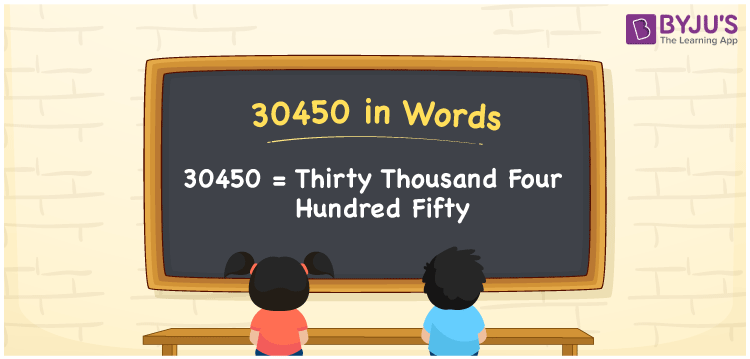# 30450 in Words

30450 in words is thirty thousand four hundred fifty. The number name of 30450 can be written using the place value system. The numeral 30450 is a natural number and exists between 30449 and 30451. Also, 30450 is a cardinal number as it helps to represent the quantity of objects equivalent to thirty thousand four hundred fifty.

 30450 in Words: Thirty Thousand Four Hundred Fifty. Thirty Thousand Four Hundred Fifty in Numerical Form: 30450.

## 30450 in English Words## How to Write 30450 in Words?

The place values of 30450 up to five-digits are given below:

 Ten-thousands Thousands Hundreds Tens Ones 3 0 4 5 0

The expanded form of 30450 is as follows:

= 3 × Ten thousand + 0 × Thousand + 4 × Hundred + 5 × Ten + 0 × One

= 3 × 10000 + 0 × 1000 + 4 × 100 + 5 × 10 + 0 × 1

= 30000 + 400 + 50

= 30450

= Thirty thousand four hundred fifty

Hence, 30450 in words is thirty thousand four hundred fifty.

30450 in words – Thirty thousand four hundred fifty

Is 30450 an odd number? – No

Is 30450 an even number? – Yes

Is 30450 a perfect square number? – No

Is 30450 a perfect cube number? – No

Is 30450 a prime number? – No

Is 30450 a composite number? – Yes

## Frequently Asked Questions on 30450 in Words

Q1

### How to spell 30450 in words?

30450 in words is thirty thousand four hundred fifty.

Q2

### Simplify 30000 + 450, and express it in words.

Simplifying 30000 + 450, we get 30450. Hence, 30450 in words is thirty thousand four hundred fifty.

Q3

### Is 30450 an even number?

Yes, 30450 is an even number.